Saturday, July 11, 2020
Home > CBSE Class 12 > NCERT Solutions for Class 12 Maths 3D Geometry Miscellaneous Exercise

# NCERT Solutions for Class 12 Maths 3D Geometry Miscellaneous Exercise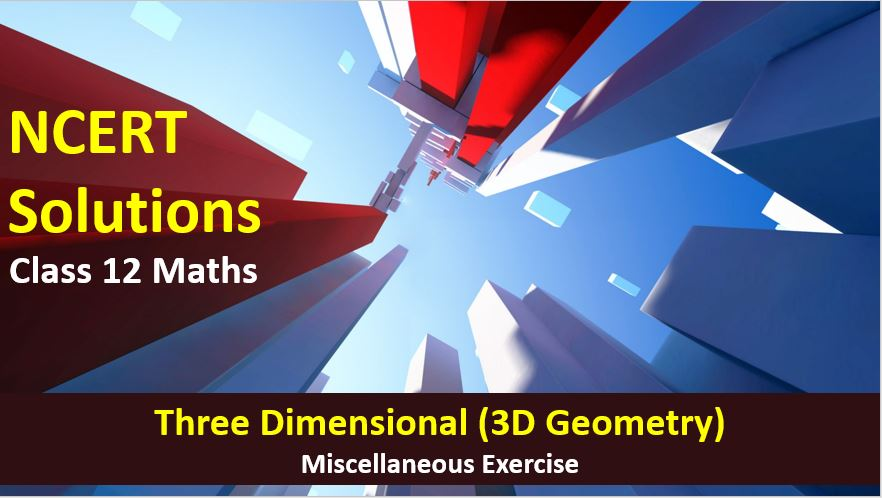# NCERT Solutions for Class 12 Maths 3D Geometry Miscellaneous Exercise

Hi Students, Welcome to Amans Maths Blogs (AMB). In this post, you will get the NCERT Solutions for Class 12 Maths 3D Geometry Miscellaneous Exercise.

CBSE Class 12th is an important school class in your life as you take some serious decision about your career. And out of all subjects, Maths is an important and core subjects. So CBSE NCERT Solutions for Class 12th Maths is major role in your exam preparation as it has detailed chapter wise solutions for all exercise. This NCERT Solutions can be downloaded in PDF file. The downloading link is given at last.

NCERT Solutions for class 12 is highly recommended by the experienced teacher for students who are going to appear in CBSE Class 12 and JEE Mains and Advanced and NEET level exams. Here You will get NCERT Solutions for Class 12 Maths 3D Geometry Miscellaneous Exercise of all questions given in NCERT textbooks of class 12 in details with step by step process.

NCERT Solutions for Class 12 Maths are not only the solutions of Maths exercise but it builds your foundation of other important subjects. Getting knowledge of depth concept of CBSE Class 12th Maths like Algebra, Calculus, Trigonometry, Coordinate Geometry help you to understand the concept of Physics and Physical Chemistry.

As we know that all the schools affiliated from CBSE follow the NCERT books for all subjects. You can check the CBSE NCERT Syllabus. Thus, NCERT Solutions helps the students to solve the exercise questions as given in NCERT Books

The PDF books of NCERT Solutions for Class 12 are the first step towards the learning and understanding the each sections of Maths, Physics, Chemistry, Biology as it all help in engineering medical entrance exams. To solve it, you just need to click on download links of NCERT solutions for class 12.

## NCERT Solutions for Class 12 Maths 3D Geometry Miscellaneous Exercise

NCERT Solutions Class 12 Math 3D Geometry Miscellaneous Exercise Ques No 1

Show that the line joining the origin to the point (2, 1, 1) is perpendicular to the line determined by the points (3, 5, – 1), (4, 3, – 1).

NCERT Solutions:

The direction ratio of the line joining origin O(0, 0, 0) to the point P(2, 1, 1) are

<(2 – 0), (1 – 0), (1 – 0)> ⇒ <2, 1, 1>

The direction ratio of the line joining A(3, 5, – 1) to the point B(4, 3, – 1) are

<(4 – 3), (3 – 5), (– 1 + 1)> ⇒ <1, –2, 0>

Now, (2)(1) + (1)(–2) + (1)(0) = 2 – 2 = 0.

Thus, OP is perpendicular to AB.

NCERT Solutions Class 12 Math 3D Geometry Miscellaneous Exercise Ques No 2

If l1, m1, n1 and l2, m2, n2 are the direction cosines of two mutually perpendicular lines, show that the direction cosines of the line perpendicular to both of these are m1n2 − m2n1 , n1l2 − n2l1 , l1m2 − l2m1.

NCERT Solutions:

Let <l, m, n> be the direction cosines of the line, which is perpendicular to two given lines whose direction cosines are <l1, m1, n1> and <l2, m2, n2>. Then,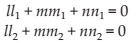Solving these two equations by cross-multiplication method, we get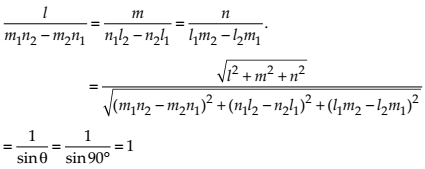Thus, the required direction cosines are l = m1n2 − m2n1 , m = n1l2 − n2l1 ,n = l1m2 − l2m1.

NCERT Solutions Class 12 Math 3D Geometry Miscellaneous Exercise Ques No 3

Find the angle between the lines whose direction ratios are a, b, c and b – c, c – a, a – b.

NCERT Solutions:

The direction cosines of the two lines are a, b, c and b – c, c – a, a – b.

Since a(b – c) + b(c – a) + c(a – b) = ab – ac + bc – ba + ac – cb = 0, then the both lines are perpendicular.

Thus, the angle between the lines is 90 degree.

NCERT Solutions Class 12 Math 3D Geometry Miscellaneous Exercise Ques No 4

Find the equation of a line parallel to x-axis and passing through the origin.

NCERT Solutions:

The line is passing through the x-axis and the parallel to the x-axis. It means, the line is x-axis itself.

The equation of the x-axis is y = 0, z = 0.NCERT Solutions Class 12 Math 3D Geometry Miscellaneous Exercise Ques No 5

If the coordinates of the points A, B, C, D be (1, 2, 3), (4, 5, 7), (– 4, 3, – 6) and (2, 9, 2) respectively, then find the angle between the lines AB and CD.

NCERT Solutions:

The direction ratios of the line joining A(1, 2, 3) to the point B(4, 5, 7) are

<(4 – 1), (5 – 2), (7 – 3)> ⇒ <3, 3, 4>

The direction ratios of the line joining C(– 4, 3, – 6) to the point D(2, 9, 2) are

<(2 + 4), (9 – 3), (2 + 6)> ⇒ <6, 6, 8>

Since the direction ratios of the both the lines are proportional, the lines AB and CD are parallel. Hence the angle between them is 0o.

NCERT Solutions Class 12 Math 3D Geometry Miscellaneous Exercise Ques No 6

If the lines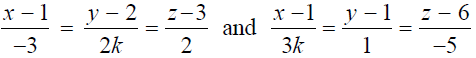are perpendicular, find the value of k.

NCERT Solutions:

The equation of the given lines areDirection ratios of the equation (1) are <-3, 2k, 2>.

Direction ratios of the equation (2) are <3k, 1, -5>.

Since the given lines are perpendicular, then

(-3)(3k) + (2k)(1) + (2)(-5) = 0 ⇒ -9k + 2k – 10 = 0 ⇒ k = -10/7

NCERT Solutions Class 12 Math 3D Geometry Miscellaneous Exercise Ques No 7

Find the vector equation of the line passing through (1, 2, 3) and perpendicular to the plane r . (i + 2 j − 5k) + 9 = 0.

NCERT Solutions:

The equation of the given plane are r . (i + 2 j − 5k) + 9 = 0.

The direction ratio of the normal to the plane is <1, 2, -5>.

Now, the line is perpendicular to the plane, it mean the line is parallel to the normal.

The direction ratio of the line is same as the direction ratio of the normal to the plane, <1, 2, -5>.

Now, the line is passing through the point (1, 2, 3) and having the direction ratio as <1, 2, -5>.

Thus, its equation is r = (i + 2 j + 3k) + λ(i + 2 j − 5k).

NCERT Solutions Class 12 Math 3D Geometry Miscellaneous Exercise Ques No 8

Find the equation of the plane passing through (a, b, c) and parallel to the plane r ⋅ (i + j + k) = 2.

NCERT Solutions:

The equation of a plane which is parallel to plane r ⋅ (i + j + k) = 2 is plane r ⋅ (i + j + k) = λ.

Since this plane is passing through the point P(a, b, c), so put r = ai + bj + ck.

Then (ai + bj + ck) ⋅ (i + j + k) = λ ⇒ λ = a + b + c.

Thus, the vector equation of the plane is r ⋅ (i + j + k) = (a + b + c).

Now, put r = xi + yj + zk, we get the cartesian equation of the plane as x + y + z = a + b + c.

NCERT Solutions Class 12 Math 3D Geometry Miscellaneous Exercise Ques No 9

Find the shortest distance between lines r = 6i + 2j + 2k + λ(i − 2j + 2k) and r = − 4ik + μ (3i − 2j − 2k).

NCERT Solutions:

From the given equation of line r = 6i + 2j + 2k + λ(i − 2j + 2k),

we have a1 = 6i + 2j + 2k and b1 = i − 2j + 2k

And, from line r = − 4ik + μ(3i − 2j − 2k),

we have a2 = − 4ik and b2 = 3i − 2j − 2k.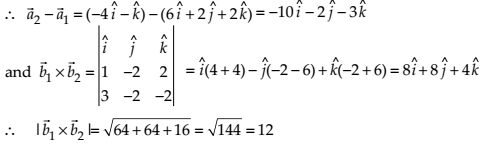Thus, shortest distance of the given two lines is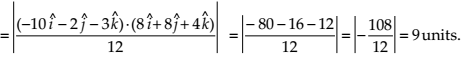NCERT Solutions Class 12 Math 3D Geometry Miscellaneous Exercise Ques No 10

Find the coordinates of the point where the line through (5, 1, 6) and (3, 4,1) crosses the YZ-plane.

NCERT Solutions:

The equation of the line passing through the points A(5, 1, 6) and B(3, 4, 1) isA point on the plane (1) is (5 – 2k, 1 + 3k, 6 – 5k). Now this line intersects YZ plane.

Then, x-coordinate = 0 ⇒ 5 – 2k = 0 ⇒ k = 5/2.

Put k = 5/2 in (5 – 2k, 1 + 3k, 6 – 5k), we get the required point as (0, 17/2, -13/2).

NCERT Solutions Class 12 Math 3D Geometry Miscellaneous Exercise Ques No 11

Find the coordinates of the point where the line through (5, 1, 6) and (3, 4, 1) crosses the ZX-plane.

NCERT Solutions:

The equation of the line passing through the points A(5, 1, 6) and B(3, 4, 1) isA point on the plane (1) is (5 – 2k, 1 + 3k, 6 – 5k). Now this line intersects ZX plane.

Then, y-coordinate = 0 ⇒ 1 + 3k = 0 ⇒ k = -1/3.

Put k = -1/3 in (5 – 2k, 1 + 3k, 6 – 5k), we get the required point as (17/3, 0, 23/3).

NCERT Solutions Class 12 Math 3D Geometry Miscellaneous Exercise Ques No 12

Find the coordinates of the point where the line through (3, – 4, – 5) and (2, – 3, 1) crosses the plane 2x + y + z = 7.

NCERT Solutions:

The equation of the line passing through the points A(3, – 4, – 5) and B(2, – 3, 1) isA point on the plane (1) is (3 – k, –4 + k, –5 + 6k).

Now, this point lies on the plane 2x + y + z = 7. Then,

2(3 – k) + (–4 + k) + (–5 + 6k) = 7 ⇒ k = 2

Put k = 2 in (3 – k, –4 + k, –5 + 6k), we get required point as (1, –2, 7).

NCERT Solutions Class 12 Math 3D Geometry Miscellaneous Exercise Ques No 13

Find the equation of the plane passing through the point (– 1, 3, 2) and perpendicular to each of the planes x + 2y + 3z = 5 and 3x + 3y + z = 0.

NCERT Solutions:

The equation of the plane passing through the points A(– 1, 3, 2) is

a(x + 1) + b(y – 3) + c(z – 2) = 0        …(1)

Now, this plane is perpendicular the planes x + 2y + 3z = 5 and 3x + 3y + z = 0, then

a + 2b + 3c = 0 and

3a + 3b + c = 0

Solving these two equations, we getPutting a = –7, b = 8 and c = –3 in the equation (1), we get

(–7)(x + 1) + (8)(y – 3) + (–3)(z – 2) = 0 ⇒ 7x – 8y + 3z + 25 = 0

NCERT Solutions Class 12 Math 3D Geometry Miscellaneous Exercise Ques No 14

If the points (1, 1, p) and (– 3, 0, 1) be equidistant from the plane r ⋅ (3i + 4j −12k) +13 = 0, then find the value of p.

NCERT Solutions:

The vector equation of the plane is r ⋅ (3i + 4j −12k) +13 = 0.

Now, put r = xi + yj + zk we get the cartesian equation of the plane as

(xi + yj + zk) ⋅ (3i + 4j − 12k) +13 = 0 ⇒ 3x + 4y − 12z + 13 = 0.

Since the given points (1, 1, p) and (– 3, 0, 1) are equidistant from this plane, then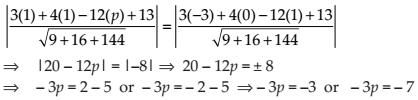Thus, p = 1 or 7/3

NCERT Solutions Class 12 Math 3D Geometry Miscellaneous Exercise Ques No 15

Find the equation of the plane passing through the line of intersection of the planes r ⋅ (i + j + k) =1  and r ⋅ (2i + 3jk) + 4 = 0 and parallel to x-axis.

NCERT Solutions:

The vector equation of the plane is r ⋅ (i + j + k) =1  and r ⋅ (2i + 3jk) + 4 = 0.

The Cartesian equation of the planes are x + y + z − 1 = 0 and 2x + 3y − z + 4 = 0.

Now, the equation of the plane passing through the intersection of the given planes is

(x + y + z − 1) + k(2x + 3y − z + 4) = 0

⇒ (1 + 2k)x + (1 + 3k)y + (1 − k)z − (1 − 4k) = 0    …(1)

This plane is parallel to x-axis, it means the normal to the plane is perpendicular to the x-axis. Then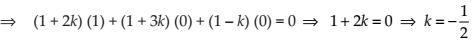Putting k = −1/2 in equation (1), we get the required equation as

⇒ (0)x + (1 − 3/2)y + (1 + 1/2)z − (1 + 2) = 0

⇒ (− 1/2)y + (3/2)z − (3) = 0

⇒ y − 3z + 6 = 0

NCERT Solutions Class 12 Math 3D Geometry Miscellaneous Exercise Ques No 16

If O be the origin and the coordinates of P be (1, 2, – 3), then find the equation of the plane passing through P and perpendicular to OP.

NCERT Solutions:

Since the direction cosine of OP is <1 – 0, 2 – 0, –3 – 0> ⇒ <1, 2, – 3>.

Now, the equation of the plane through the plane P and perpendicular OP is

(1)(x – 1) + (2)(y – 2) + (–3)(z + 3) = 0

⇒ x – 1 + 2y – 4 – 3z – 9 = 0

⇒ x + 2y – 3z – 14 = 0

NCERT Solutions Class 12 Math 3D Geometry Miscellaneous Exercise Ques No 17

Find the equation of the plane which contains the line of intersection of the planes r ⋅ (i + 2j + 3k) − 4 = 0, r ⋅ (2i + jk) + 5 = 0 and which is perpendicular to the plane r ⋅ (5i + 3j − 6k) + 8 = 0.

NCERT Solutions:

Given equations of the planes are

r ⋅ (i + 2j + 3k) − 4 = 0 ⇒ x + 2y + 3z – 4 = 0         …(1)

r ⋅ (2i + jk) + 5 = 0 ⇒ 2x + y – z + 5 = 0             …(2)

Any plane passing through the intersection of the planes (1) and (2) is

(x + 2y + 3z – 4) + k(2x + y – z + 5) = 0

⇒ (1 + 2k)x + (2 + k)y + (3 – k)z + (5k – 4) = 0     …(4)

Now, this plane is perpendicular to the plane r ⋅ (5i + 3j − 6k) + 8 = 0 ⇒ 5x + 3y − 6y + 8 = 0.

Thus, we have

⇒ 5(1 + 2k) + 3(2 + k) – 6(3 – k) = 0

⇒ k = 7/19

Putting k = 7/19 in equation (4), we get required equation of plane as 33x + 45y + 50z – 41 = 0.

NCERT Solutions Class 12 Math 3D Geometry Miscellaneous Exercise Ques No 18

Find the distance of the point (– 1, – 5, – 10) from the point of intersection of the line r = 2i − j + 2k + λ(3i + 4j + 2k) and the plane r ⋅ (i − j + k) = 5.

NCERT Solutions:NCERT Solutions Class 12 Math 3D Geometry Miscellaneous Exercise Ques No 19

Find the vector equation of the line passing through (1, 2, 3) and parallel to the planes r ⋅ (ij + 2k) = 5 and r ⋅ (3i + j + k) = 6.

NCERT Solutions:NCERT Solutions Class 12 Math 3D Geometry Miscellaneous Exercise Ques No 20

Find the vector equation of the line passing through the point (1, 2, – 4) and perpendicular to the two lines:NCERT Solutions:NCERT Solutions Class 12 Math 3D Geometry Miscellaneous Exercise Ques No 21

Prove that if a plane has the intercepts a, b, c and is at a distance of p units from the origin, thenNCERT Solutions:NCERT Solutions Class 12 Math 3D Geometry Miscellaneous Exercise Ques No 22

Distance between the two planes: 2x + 3y + 4z = 4 and 4x + 6y + 8z = 12 is

(A) 2 units

(B) 4 units

(C) 8 units

(D) 2/Root(29) units

NCERT Solutions: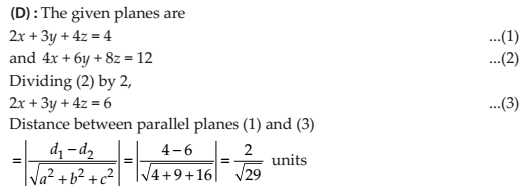NCERT Solutions Class 12 Math 3D Geometry Miscellaneous Exercise Ques No 23

The planes: 2x – y + 4z = 5 and 5x – 2.5y + 10z = 6 are

(A) Perpendiculars

(B) Parallel

(C) Intersect y-axis

(D) passes through (0, 0, 5/4)

NCERT Solutions:

error: Content is protected !!